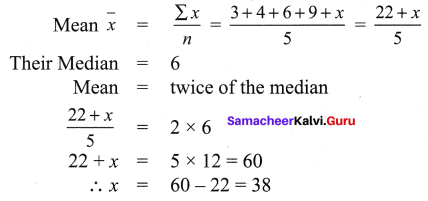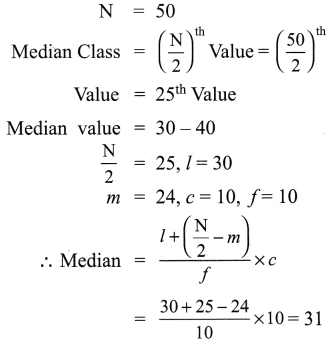# Samacheer Kalvi 9th Maths Solutions Chapter 8 Statistics Ex 8.2

## Tamilnadu Samacheer Kalvi 9th Maths Solutions Chapter 8 Statistics Ex 8.2

Question 1.
Find the median of the given values : 47, 53, 62, 71, 83, 21, 43, 47, 41.
Solution:
47, 53, 62, 71, 83, 21, 43, 47, 41
Ascending order = 21, 41, 43, 47, 47, 53, 62, 71, 83
The number of values 9, which is odd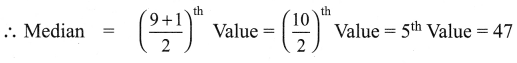Question 2.
Find the Median of the given data: 36, 44, 86, 31, 37, 44, 86, 35, 60, 51.
Solution:
36, 44, 86, 31, 37, 44, 86, 35, 60, 51
Ascending order 31, 35, 36, 37, 44, 44, 51, 60, 86, 86
The number of values = 10, which is an even number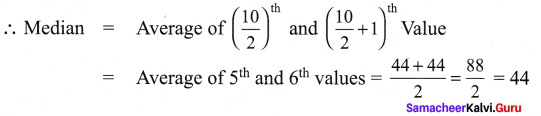Question 3.
The median of observation 11, 12,14, 18, x + 2, x + 4, 30, 32, 35, 41 arranged in ascending order is 24. Find the values of x.
Solution:
11, 12, 14, 18, x + 2, , x + 4, 30, 32, 35, 41
The number of values = 10, which is an even number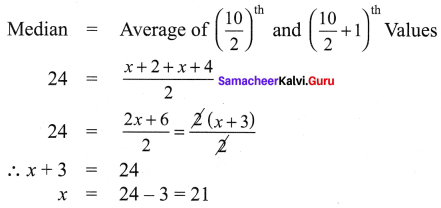Question 4.
A researcher studying the behaviour of mice has recorded the time (in seconds) taken by each mouse to locate its food by considering 13 different mice as 31, 33, 63, 33, 28, 29, 33, 27, 27, 34, 35, 28, 32. Find the median time that mice spent in searching its food.
Solution:
31, 33, 63, 33, 28, 29, 33, 27, 27, 34, 35, 28, 32 Writing in ascending order we get 27, 27, 28, 28, 29, 31, 32, 33, 33, 33, 34, 35, 63
Number of values = 13 which is an odd number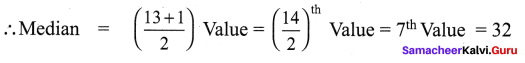Question 5.
The following are the marks scored by the students in the Summative Assessment exam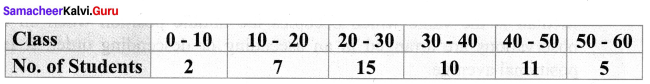Calculate the median.
Solution: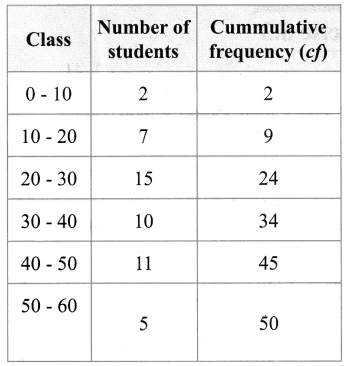Question 6.
The mean of five positive integers is twice their median. If four of the integers are 3, 4, 6, 9 and median is 6, then find the fifth integer.
Solution:
The five integers are 3, 4, 6, 9, x